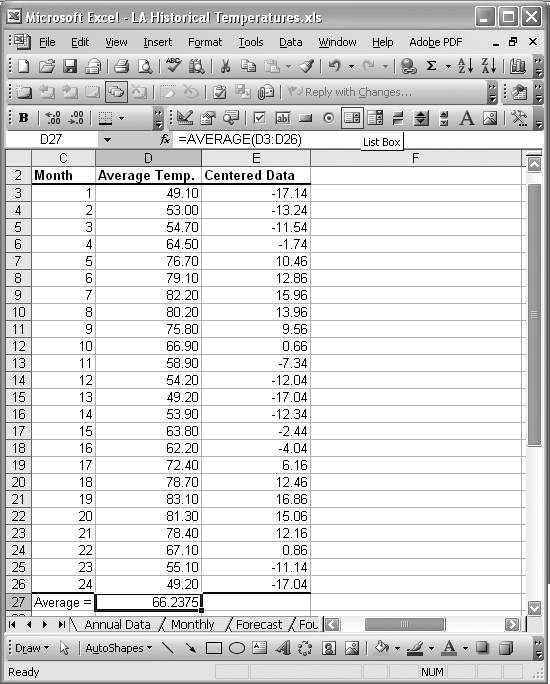# Centering Data

Problem

You need to center a data series before further analysis.

Solution

Use basic spreadsheet techniques and Excel's built-in formulas to center the data.

Discussion

Centering in this context refers to the process of removing a bias or offset in a series of data so that the resulting series values fluctuate approximately about 0. Centering is often required before processing data using forecasting techniques, and can be accomplished quite easily in Excel. There are a number of ways to center a data series, and here we'll use the standard approach of subtracting the average of the data in the series from each data item in the series.

Figure 6-17 shows an example spreadsheet I set up.

Figure 6-17. Centering exampleThe first column contains the month label, while the second column contains the original data series before centering. This series represents average monthly temperatures.

To center the data, I first computed the average of all the temperature values in the original data series. Cell D27 contains the formula =AVERAGE(D3:D26) that computes the average of all temperatures.

The column adjacent to the original data series contains the centered data (column E). The formulas in this column are of the form =D3-\$D\$27. All this does is subtract the average temperature contained in cell D27 from every temperature value in the original series. The result is a centered data series with the offset removed.

I set up a few plots so you can see the difference. Figure 6-18 shows the original data series and Figure 6-19 shows the centered data series.

Figure 6-18. Original data seriesFigure 6-19. Centered data seriesThe original data series oscillates about the average temperature up in the 60s, while the centered data series oscillates about the 0 axis.

This isn't the only way to center data. Some techniques involve computing a weighted average of the data and using the result to center the series.Excel Scientific and Engineering Cookbook (Cookbooks (OReilly))
ISBN: 0596008791
EAN: 2147483647
Year: N/A
Pages: 206
Authors: David M Bourg